Home > CC4 > Chapter A > Lesson A.1.8 > ProblemA-86

A-86.

Simplify each expression. Homework Help ✎

1.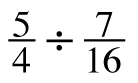2.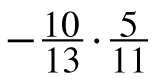3.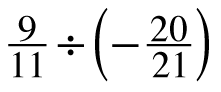4.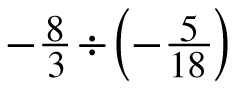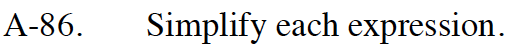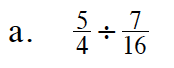When you divide a fraction, you arealso multiplying by its reciprocal.

$\frac{5}{4}\cdot \frac{16}{7}$

$\frac{20}{7}=\ 2 \frac{6}{7}$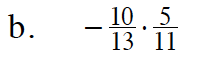Multiply.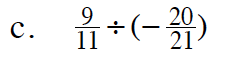See part (a).

$\frac{9}{11}\cdot \left(-\frac{21}{20}\right)$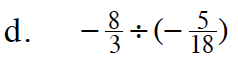See part (a).

$\frac{144}{15}$

Remember to reduce the fraction and convert to a mixed number.